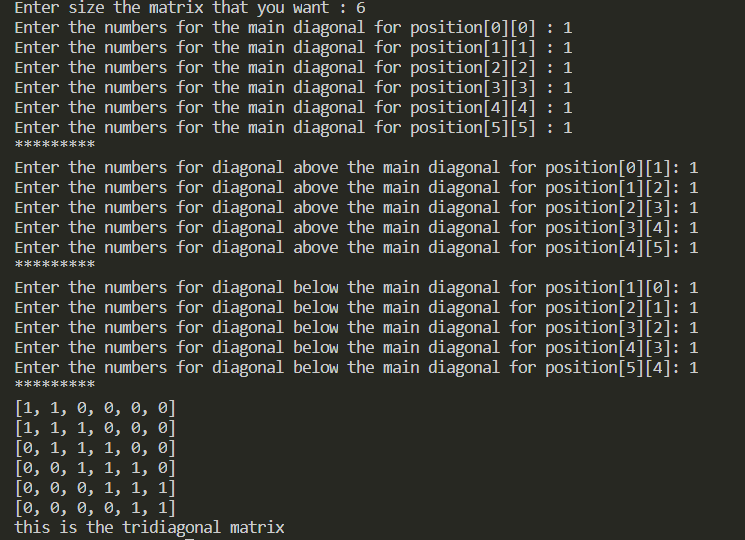Related Articles
Tridiagonal matrix in python
• Difficulty Level : Hard
• Last Updated : 02 Feb, 2021

A tridiagonal matrix is a matrix that has non-zero elements only at the main diagonal, diagonal below and above it. All other elements are zero. For this reason tridiagonal matrices of dimension smaller than or equal to 3 seem meaningless.

Example 1:

[a11,  a22,  0  ,  0  ,  0  ,  0  ]

[a21,  a22,  a23,  0  ,  0  ,  0  ]

[0  ,  a32,  a33,  a34,  0  ,  0  ]

[0  ,  0  ,  a43,  a44,  a55,  0  ]

[0  ,  0  ,  0  ,  a54,  a55,  a56]

[0  ,  0  ,  0  ,  0  ,  a65,  a66]

Example 2:

[1,  1,  0,  0,  0,  0]

[1,  1,  1,  0,  0,  0]

[0,  1,  1,  1,  0,  0]

[0,  0,  1,  1,  1,  0]

[0,  0,  0,  1,  1,  1]

[0,  0,  0,  0,  1,  1]

### Approach

• Take the size of the matrix as input
• Check if greater than 3
• if not, exit
• if yes, proceed further
• Take elements of the matrix as input
• Now put zero everywhere except at main diagonal and diagonals below and above the main diagonal.

Program:

## Python3

 `# if you enter number n it will automatically ``# be considered as a square matrix of size n by n``size_of_a_matrix ``=` `int``(``input``(``"Enter size the matrix that you want : "``))``  ` `if` `size_of_a_matrix <``=` `3``:``     ` `    ``# since size should be greater than 3``    ``print``(``"Please enter the size that is greater than 3"``)``    ``exit()`` ` `diagonal ``=` `[]``numbers1 ``=` `[[``0` `for` `j ``in` `range``(``0``, size_of_a_matrix)]``            ``for` `i ``in` `range``(``0``, size_of_a_matrix)]``  ` `# created a loop to enter numbers``for` `a ``in` `range``(size_of_a_matrix):``    ``numbers1 ``=` `int``(``input``(f``"Enter the numbers for the main diagonal for position[{a}][{a}] : "``))``      ` `    ``# appending the values to the list``    ``diagonal.append(numbers1)`` ` `diagonalAbove ``=` `[]``print``(``"*********"``)``  ` `# created a loop to enter numbers``for` `k ``in` `range``(size_of_a_matrix``-``1``):``    ``numbers2 ``=` `int``(``input``(f``"Enter the numbers for diagonal above the main diagonal for position[{k}][{k+1}]: "``))``     ` `    ``# appending the values to the list``    ``diagonalAbove.append(numbers2)`` ` `diagonalBelow ``=` `[]``print``(``"*********"``)``  ` `# created a loop to enter numbers``for` `z ``in` `range``(size_of_a_matrix``-``1``):``    ``numbers3 ``=` `int``(``input``(f``"Enter the numbers for diagonal below the main diagonal for position[{z+1}][{z}]: "``))``     ` `    ``# appending the values to the list``    ``diagonalBelow.append(numbers3)``print``(``"*********"``)``  ` `  ` `def` `tridiagonal(size_of_a_matrix, diagonal, diagonalAbove, diagonalBelow):``  ` `    ``matrix ``=` `[[``0` `for` `j ``in` `range``(size_of_a_matrix)]``              ``for` `i ``in` `range``(size_of_a_matrix)]``     ` `    ``for` `k ``in` `range``(size_of_a_matrix``-``1``):``        ``matrix[k][k] ``=` `diagonal[k]``        ``matrix[k][k``+``1``] ``=` `diagonalAbove[k]``        ``matrix[k``+``1``][k] ``=` `diagonalBelow[k]``     ` `    ``matrix[size_of_a_matrix``-``1``][size_of_a_matrix ``-` `1``] ``=` `diagonal[size_of_a_matrix``-``1``]``  ` `    ``# so that the values will print row by row``    ``for` `row ``in` `matrix:``        ``print``(row)`` ` `    ``return` `"this is the tridiagonal matrix"`` ` `# printing final values``print``(tridiagonal(size_of_a_matrix, diagonal, diagonalAbove, diagonalBelow))`

Output:output of our program

Attention geek! Strengthen your foundations with the Python Programming Foundation Course and learn the basics.

To begin with, your interview preparations Enhance your Data Structures concepts with the Python DS Course.

My Personal Notes arrow_drop_up